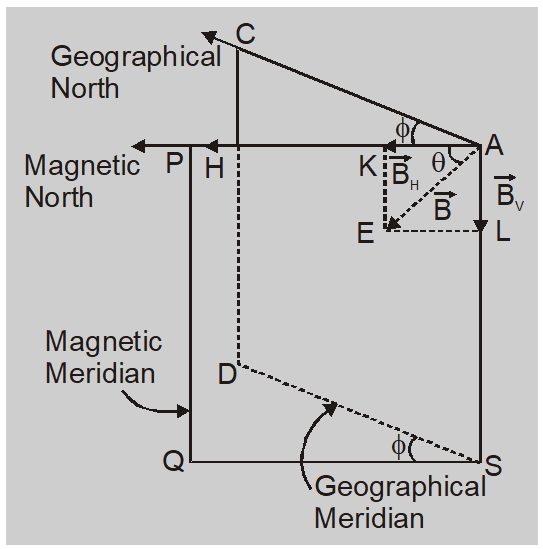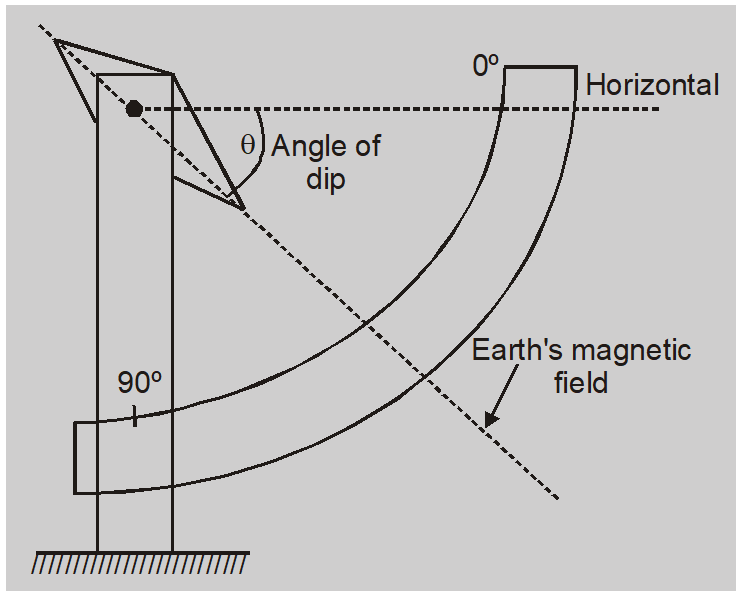# Magnetic Elements - Magnetism Class 12 || Physics Notes

The physical quantities which determine the intensity of earth's total magnetic field completely both in magnitude and direction are called magnetic elements.

### Angle of Declination $(\phi)$:

The angle between the magnetic meridian and geographic meridian at a place is called angle of declination.

(a) Isogonic Lines : Lines drawn on a map through places that have same declination are called isogonic lines.

(b) Agonic Line : The line drawn on a map through places that have zero declination is known as an agonic line.

###Angle of Dip or Inclination

The angle through which the N pole dips down with reference to horizontal is called the angle of dip. At magnetic north and south pole angle of dip is $90^{\circ}$. At magnetic equator the angle of dip is zero.

OR The angle which the direction of resultant field of earth makes with the horizontal line of magnetic meridian is called angle of dip.(a) Isoclinic Lines : Lines drawn up on a map through the places that have same dip are called isoclinic lines.

(b) Aclinic Line : The line drawn through places that have zero dip is known as aclinic line. This is the magnetic equator.

### Horizontal component of earth's magnetic field

The total intensity of the earth's magnetic field makes an angle  with horizontal. It has

(i) Component in horizontal plane called horizontal component $B_{H}$.

(ii) Component in vertical plane called vertical component $B_{V}$

$B _{ V }= B \sin \theta \quad B _{ H }= B \cos \theta$

So $\frac{ B _{ V }}{ B _{ H }}=\tan \theta \quad$ and $\quad B =\sqrt{ B _{ H ^{2}}+ B _{ V ^{2}}}$

#### IMPORTANT POINTS

1. If $\theta$ and $\phi$ are known we can find direction of B.
2. If $\theta$ and $B_{H}$ are known we can find magnitude of B.
3. So if $\theta$, $\phi$ and $B_{H}$ are known we can find total field at a place. So these are called as Elements of earth's magnetic field.
4. The declination gives the plane, dip gives the direction and horizontal component gives magnitude of earth's magnetic field.
5. If declination is ignored, then the horizontal component of earth's magnetic field is from geogrophic south to geographic north.
6. Angle of dip is measured by instrument called dip circle.
Also Read: Biot Savart's Law   Click here for the Video tutorials of Magnetic Effect of Current Class 12
About eSaral At eSaral we are offering a complete platform for IIT-JEE & NEET preparation. The main mission behind eSaral is to provide education to each and every student in India by eliminating the Geographic and Economic factors, as a nation’s progress and development depends on the availability of quality education to each and every one. With the blend of education & technology, eSaral team made the learning personalized & adaptive for everyone.